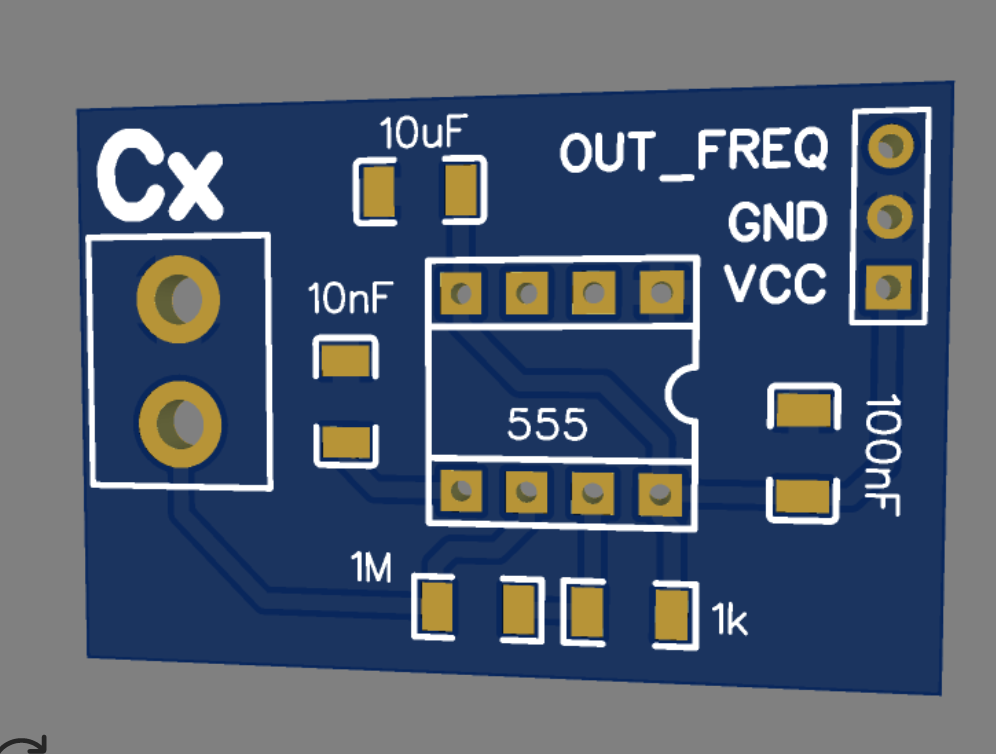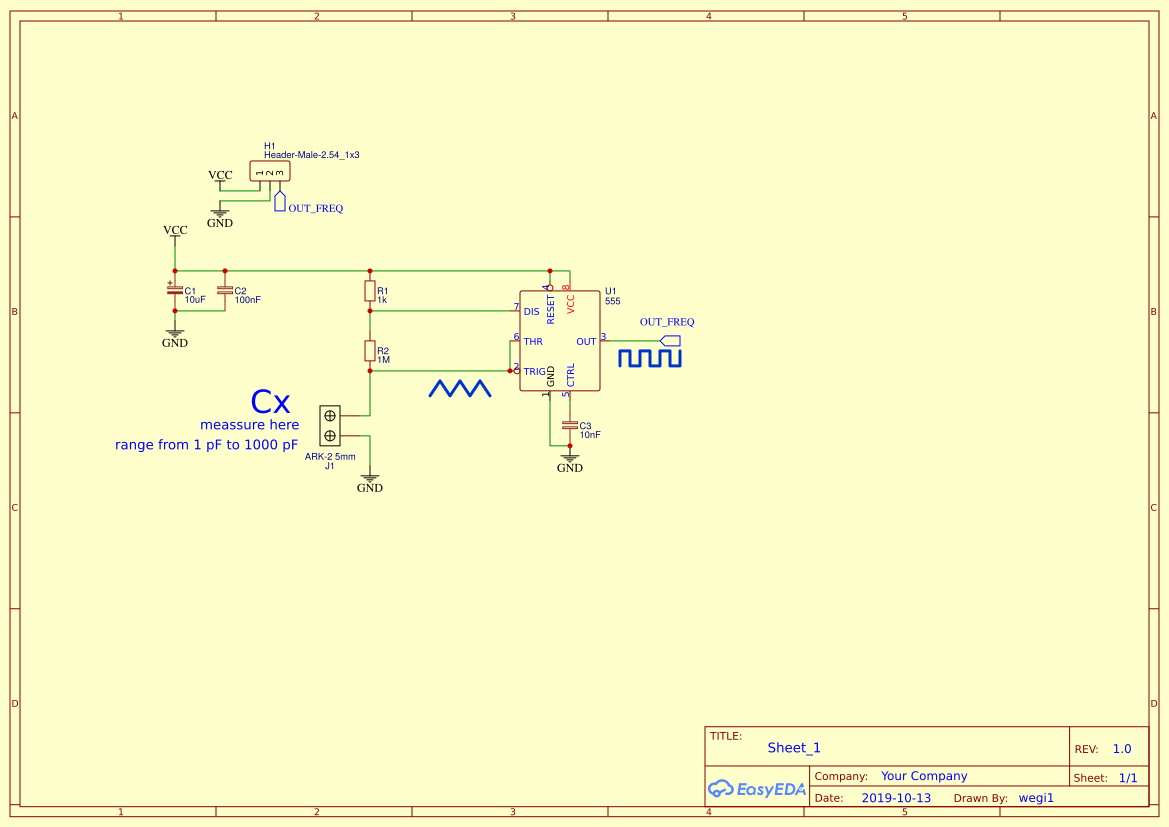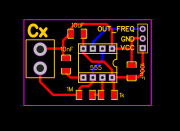Public Domain

##### Tags
diy home diy 555 measure
Recommend Projects

# 555_meassure_cappacitance_by_frequency

7 months ago 211
• Description
• Documents
• BOM
• Attachments
• Members

## Description# Using 555 as a multivibrator we can measure the capacity of the connected capacitor.

At all times, the 555 charges to 2/3 VCC and discharges to 1/3 VCC the connected capacitor, supplying a rectangular signal at the output in accordance with the capacitor charging and discharging cycle.

In this system, by measuring the frequency of the output signal, we can calculate the capacitance of the connected capacitor. The frequency of the output signal is determined by the equation - to increase the readability of the expression, I added square brackets:

f = [1/ln(2)] / [(R1+R2+R2)*C]

where ln means natural logarithm f - frequency [Hz] - output square wave signal R - resistance [Ohm] - R1, R2 resistors C - capacitance [F] - C capacitor

It is noteworthy that the above equation is characterized by a linear relationship. In other words, the output frequency is proportional to the capacitance.

And for example if:

R1 = 1000 [Ohm] R2 = 1 000 000 [Ohm]

By substituting the following values for the formula for C, we obtain the following results IF

C = :

1 [pf] - output frequency = 720987 [Hz] 2 [pf] - output frequency = 360494 [Hz] 10 [pf] - output frequency = 72099 [Hz] ... 100 [pf] - output frequency = 7210 [Hz] 101 [pf] - output frequency = 7138 [Hz]

Assuming that the system itself has parasitic capacities, examples 5 and 6 show how they can be compensated. That is, from the calculated value, you must subtract the own capacity of the circuit. (we are talking about measuring very small capacities).

If we know the values of the connected resistors and the output frequency, then by translating the above equation we can calculate the attached capacitance.

C = [1/ln(2)] / [(R1+R2+R2)*f]

By the way: 1/ln(2) = approximately 1,44 So the above equation can therefore be represented as a rounding:

C = 1,44 / (R1+2R2)f

So now the reverse. Using the above formula, knowing the values of R1, R2 and frequency, we calculate the attached capacity.

output frequency = 720987 [Hz] C = 1 [pf] output frequency = 360494 [Hz] C = 2 [pf] output frequency = 72099 [Hz] C = 10 [pf] ... output frequency = 7210 [Hz] C = 100 [pf] output frequency = 7138 [Hz] C = 101 [pf]

## To know the size of the circuit's own capacity, simply measure the frequency of the output signal without a capacitor connected. Or even better - connect one tiny 10-15 [pF] capacitor, which will collect parasitic capacitances in parallel.

So let's just hypothetically assume that the own capacity of the circuit is 100 [pF], the obtained frequency measurement indicates the value 101 [pF], so the capacity of the connected capacitor is:

measured capacity MINUS own capacity of the circuit EQUAL capacitance of the connected capacitor

Therefore

101 [pF] - 100 [pF] = 1 [pF]

# By changing the R1,R2 resistor values, you can change the measurement range of the capacitance.

To filter the power, 10 [uF] and 100 [nF] capacitors were added. A capacitor of 10 [nF] on a CTRL pin was also added for stabilization.

I forgot to add that 555 has this feature that the output frequency value is independent of the VCC level. The capacitor is charged to 2/3 VCC and discharged to 1/3 VCC. This property 555 is of great importance when matching the voltage levels of the output signal to peripheral systems.

For example HI output state when VCC = 5V is typically 3.3V (no less than 2.75 V)## Sheet_1## 555_MEASSURE_CAPPACITANCE_BY_FREQUENCY_PCB## BOM

ID Name Designator Footprint Quantity
1 1k R1 R1206 1
2 1M R2 R1206 1
3 10uF C1 C1206 1
4 555 U1 DIL08 1
6 ARK-2 5mm J1 ARK-2 5MM 1
7 100nF C2 C1206 1
8 10nF C3 C1206 1

## Attachments

None
Success
The owner does not allow comments in this project now